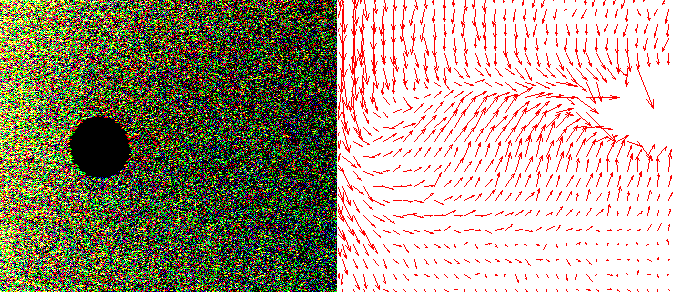## Apr 10, 2010

### Fluid dynamics with cellular automata on a GPU## 1. Introduction

This is going to be an another cellular automata related post. I will describe so called FHP GAS automaton on a hexagonal grid and document my attempts on (loosely) re-creating the result from Salem/Wolfram article on fluid dynamics. In contrast with my previous posts on a similar topic, I won't go into deeper details of GPU implementation here. Instead, I'm going to just share a couple of screenshots of what I've got so far.

Here is what we will be trying to re-create:

Figure 2. Flow past an obstacle (screenshot from the aforementioned Salem/Wolfram work)

What's interesting in all this is that we can observe macro effects (such as flow around an obstacle), while modeling the system on a micro-level only, as every particle interaction is strictly localized.

## 2. FHP GAS cellular automata

The cellular automaton we are going to use to model fluid dynamics, FHP GAS, is defined as follows:

• The CA operates on a hexagonal grid and uses the moore neighborhood.

• There are 6 directions, in which particles are moving.

• Every cell can hold up to 6 particles moving in different directions.

• When a cell holds several particles, a collision might occur.

• A collision changes the moving directions of colliding particles, but total energy and momentum remain constant.

This is very similar to the TM GAS described earlier. It uses the hexagonal grid though, so there are more possibilities for energy/momentum conserving collisions (TM GAS has only one, obviously). Also, FHP GAS uses moore neighborhood (unlike TM GAS, which is defined on margolus neighborhood).

To model hexagonal moore neighborhood, I use the "bricks" technique I used for Belousov-Zhabotinsky modeling. Since every hexagonal cell consists of two pixels, and each pixels is a 4-vector, it's easy to encode 6 different states in one cell. For example, let left pixel encode ```(UL, UR, DL, DR)``` directions, and right one `(L, R, n/a, n/a)`. Thus, a single hexagonal cell containing particles moving in all 6 directions would be encoded as ```(1, 1, 1, 1) (1, 1, 0, 0)```.

Note that this is different from moore neighborhood representation we used for Belousov-Zhabotinsky automaton, because here two halves of one hexagonal cell could very well be different. This requires some changes in handling of neighbors in the fragment shader.

Figure 3. Subsequent steps (left to right) of a 3-way collision

The figure above shows an example of a collision of three particles. Total momentum is zero before and after the collision. Middle fragment of the picture shows 2nd and 3rd steps of simulation together (hollow circles depict the state before collision, i.e. on the 2nd step).

And here is one possible way of four particles to collide:

Another possible outcome of a potential collision is skipping, i.e. particles go through each other freely. When a collision occurs, one could choose the outcome by applying them randomly, but in order for the CA to remain deterministic, it's better to rotate possible collision outcomes in a round-robin fashion.

Now, let's also introduce impenetrable walls, which would act as a mirror when a particle collides with it. We can use `'B'` component of the right pixel of a hexagonal cell to encode the walls.

Finally, we can artificially add an external flow of particles by adding right-moving particles on the left boundary, and removing such particles reaching right boundary. We need the flow to get a flow-aroung-obstacle picture.

## 3. Building vector field

In order to construct a vector field for the simulated gas, I used a rectangular grid over the whole texture. For each step of the simulation, we can build a 2D vector for every grid cell, based on the number of particles moving in every direction in the cell. It also makes sense to smooth things a little bit by averaging said vector over several simulation steps.

Obtained vector field snapshots can be visualised easily using gnuplot software.

## 4. Results so far

Though I haven't reproduced obstacle runaround results accurately (possible because I use slightly different method of building vector field, comparing to the Toffoli & Margolus book), I've obtained some more or less interesting results for different boundary conditions and for `512x512` texture.

This section is a dumping grounds for gnuplot screenshots of these results.